# Calculation of the 4D complex number tau.

It is about high time for a new post, now some time ago I proposed looking at those old classical equations like the heat and wave equation and compare that to the Schrödinger equation. But I spilled some food on my notes and threw it away, anyway everybody can look it up for themselves; what often is referred to as the Schrödinger equation looks much more like the heat equation and not like the classical wave equation…

Why this is I don’t know.

This post is a continuation from the 26 Feb post that I wrote after viewing a video from Gerard ‘t Hooft. At the end of the 26 Feb post I showed you the numerical values for the  logarithm of the 4D number tau. This tau in any higher dimensional number system (or a differential algebra in case you precious snowflake can only handle the complex plane and the quaternions) is always important to find.

Informally said, the number tau is the logarithm of the very first imaginary component that has a determinant of 1. For example on the complex plane we have only 1 imaginary component usually denoted as i. Complex numbers can also be written as 2 by 2 matrices and as such the matrix representation of i has a determinant of 1.
And it is a well known result that log i = i pi/2, implicit the physics professors use that every day of every year. Anytime they talk about a phase shift they always use this in the context of multiplication in the complex plane by some number from the unit circle in the complex plane.

In this post, for the very first time after being extremely hesitant in using dimensions that are not a prime number, we go to 4D real space. Remark that 4 is not a prime number because it has a prime factorization of 2 times 2.

Why is that making me hesitant?
That is simple to explain: If you can find the number i from the complex plane into my freshly crafted 4D complex number system, it could very well be this breaks down to only the complex plane. In that case you have made a fake generalization of the 2D complex numbers.

So I have always been very hesitant but I have overcome this hesitation a little bit in the last weeks because it is almost impossible using the complex plane only to calculate the number tau in the four dimensional complex space…

May be in a future post we can look a bit deeper in this danger; if also Cauchy-Riemann equations are satisfied in four real variables, that would bring a bit more courage to further study of the 4D complex number system.

__________

After the introduction blah blah words I can say the 4D tau looks very beautiful. That alone brings some piece of mind. I avoided all mathematical rigor, no ant fucking but just use numerical results and turn them into analytical stuff.

That is justified by the fact that Gerard is a physics professor and as we know from experience math rigor is not very high on the list or priorities over there…

That is forgiven of course because the human brain and putting mathematical rigor on the first place is the perfect way of making no progress at all. In other sciences math should be used as a tool coming from a toolbox of reliable math tools.

__________

This post is seven pictures long, all are 550 by 775 pixels in size except for the last one that I had to make a little bit longer because otherwise you could not see that cute baby tau in the 4D complex space.

Here we go: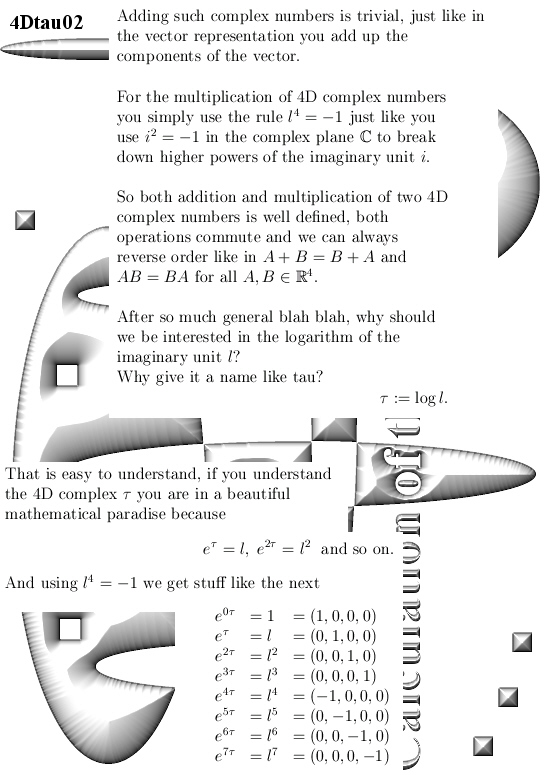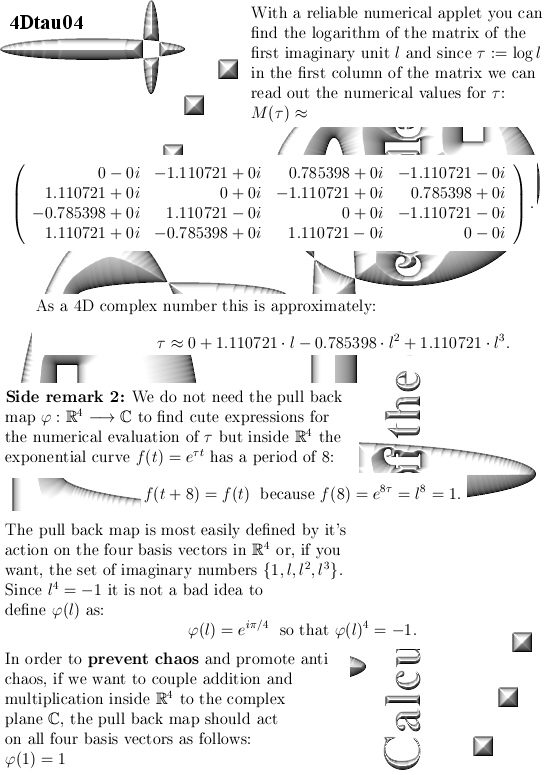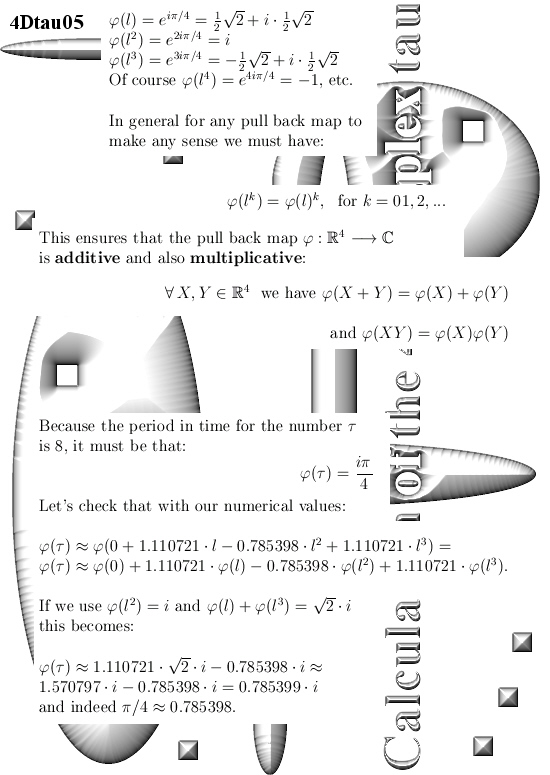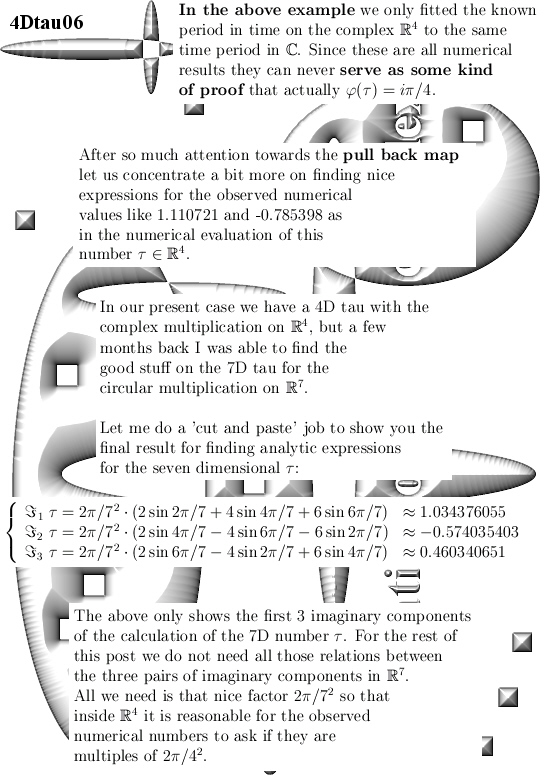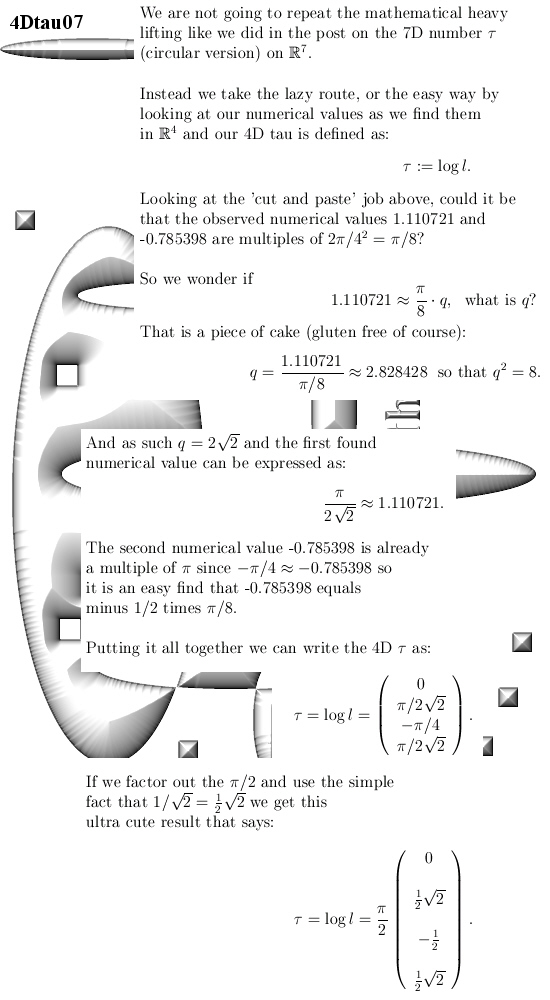Just take your time and look at this ultra cute number tau.

It is very very hard to stay inside the complex plane, of course the use of 4 by 4 matrices is also forbidden, and still find this result…

I am still hesitant about using dimensions that are not prime numbers, but this is a first result that is not bad.

End of this post.